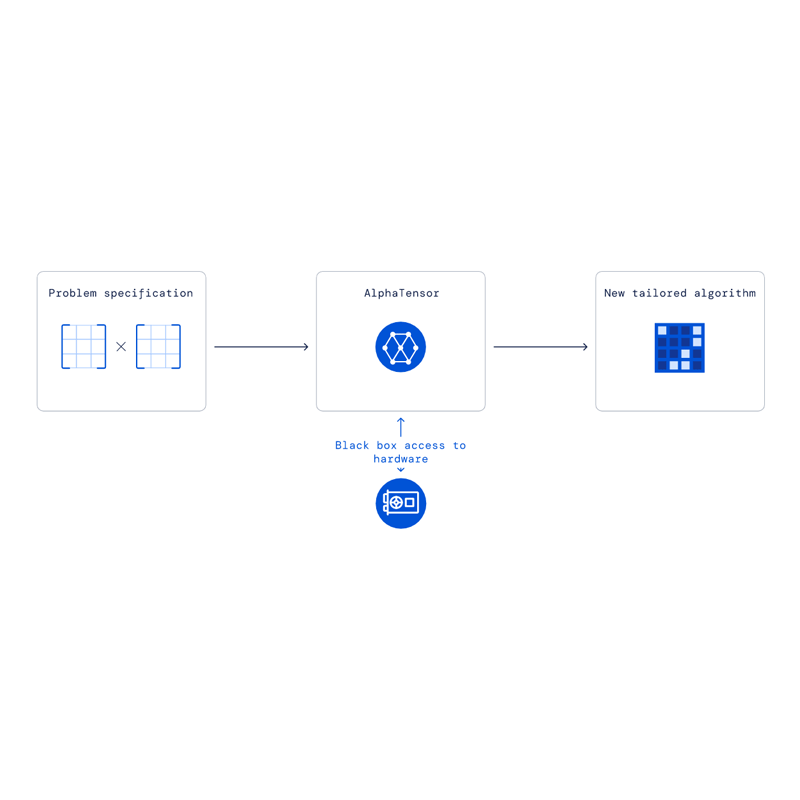# DeepMind Unveils 'AlphaTensor' AI That Is Capable Of Inventing its Own AlgorithmsWhat computers do best, is calculating. Using algorithms, computers can be made to do simple calculations, to dealing with exorbitant numbers and complex equations.

And whenever a more efficient algorithm is introduced, chances are, computers can use it to improve their calculations. And this time, it's DeepMind, the subsidiary of Google, that managed to find a way to greatly improve computers' abilities in conducting calculations.

And the company discovered the way using AI.

According to its report, DeepMind applied reinforced learning techniques to find a more efficient way to do calculate mathematical matrix multiplications, which is a type of calculation that computers can use extensively while processing data.

To do this, DeepMind used what it calls 'AlphaTensor' AI, which has been designed to purposefully discover new algorithms that can be used to solve mathematical problems.

And according to its research paper, AlphaTensor has found a method of carrying out matrix multiplications that is more efficient that some human-made algorithms that have lasted 50 years.

Founded in London in 2010, DeepMind has become famous for defeating the world champion at board game Go with its AlphaGo AI.

Since then, subsequent products also accomplished different new feats. From an AI capable of programming, and another AI that rivals OpenAI's GPT-3. an AI that can dribble a ball and carry boxes, and among others, also include an AI that teases Artificial General Intelligence.

Projects after more projects, the company also sets its sight on solving mathematical problems themselves.

And AlphaTensor is its approach.

The AI has successfully automate the discovery of algorithms which act as shortcuts when multiplying matrices.

For more than many years, mathematicians have been applying algorithms to these complex array multiplications, some of which are used in computer science.

DeepMind researcher Alhussein Fawzi and his colleagues used deep reinforcement to rediscover earlier algorithms, and also to find new ones.

And AlphaTensor here, discovered the novel method by playing a game, in which it is rewarded when it finds the best approach to multiplying two matrices.The single-player game played by AlphaTensor, where the goal is to find a correct matrix multiplication algorithm. (Credit: DeepMind)

In this case, the AI agent takes on puzzles in the form of a 3D tensor or a grid of numbers, which it must complete in the fewest moves.

Each step represents a move in solving the matrix-based puzzle, which cam contain trillions of possible moves.

Fawzi explained that mapping out the space of algorithmic discovery was already difficult, and navigating through it was even more difficult. Regardless, AlphaTensor managed to find a more efficient algorithm to solve the multiplication of matrices that is an improvement to the algorithms humans have been using for decades.

In one example, DeepMind said that the traditional algorithm taught in school multiplies a 4x5 by 5x5 matrix using 100 multiplications. Using human ingenuity, this number can be reduced to 80.

AlphaTensor here, managed to do the same operation using just 76 multiplications.

As a result of this, the algorithm can be made to multiply large matrices with speed that is 10-20% faster than the commonly used algorithms using the same hardware.Algorithm discovered by AlphaTensor uses 76 multiplications, an improvement over state-of-the-art algorithms. (Credit: DeepMind)

The researchers claim the technique could benefit computational tasks that use multiplication algorithms.

This is because matrix multiplication is commonly used for processing smartphone images, understanding speech commands, generating computer graphics for computer games, data compression and many many more.

In the modern world of technology, many use expensive GPU hardware to boost matrix multiplication efficiency.

DeepMind's solution can be useful, since the extra speed would be game-changing in terms of lowering costs and saving energy.

But more importantly, for DeepMind at least, is that the research demonstrates how reinforcement learning can be used to find new and unexpected solutions to known problems.

Published:
07/10/2022